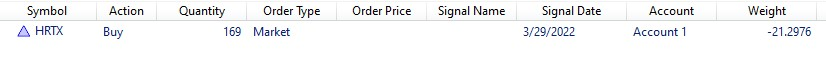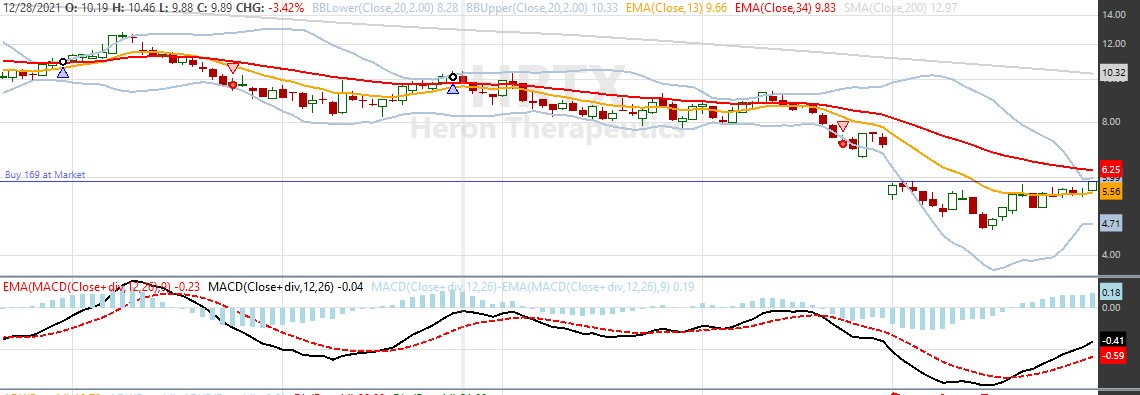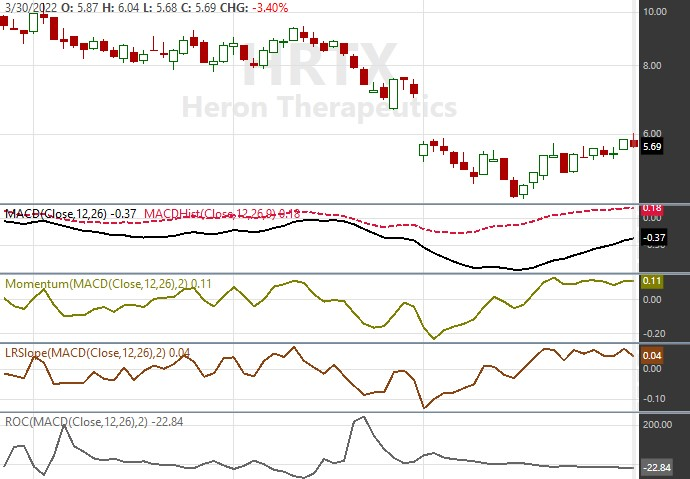I've often used ROC to calculate percent change; however I stumbled onto an issue with it -- negative values.

I stumbled onto this issue when using ROC to calculate the change in MACD as a weight for a buy which can be negative as well as positive. I saw this in the Signal view:Weight clearly shows a negative value -- indicating trending downward. That puzzled me as the chart view obviously showed the MACD trending up on the latest date.The code for the buy and assigning the weight is as follows:
```CODE:   Transaction t = PlaceTrade(bars, TransactionType.Buy, OrderType.Market);
t.Weight = ROC.Value(idx, _tsMacd, 2);
WriteToDebugLog(string.Concat("(", _tsMacd[idx].ToString("n4"), " - ", _tsMacd[idx-2].ToString("n4"), ") / ", _tsMacd[idx-2].ToString("n4"), " = ", t.Weight.ToString("n4")));
```

I added the WriteToDebugLog so I could verify what I suspected. Here's the result from the debug log:
QUOTE:
(-0.4070 - -0.5171) / -0.5171 = -21.2976

The issue here is that since this is a negative number the denominator needs to be the absolute value to get the correct percent. It doesn't appear ROC does that.

Based on what I've seen for ROC calculations, the indicator is not expected to use the absolute value for the denominator because prices are always positive. So I'm not suggesting ROC needs to be fixed. I just wanted to point out using it to get percentage change doesn't appear to work properly if negative values could be in the calculation. I've seen a few posts where ROC was expected to provide the correct percentage change, but now it appears that's only true when values are always positive.

Feel free to correct me if I'm missing something.
1
387
Solved
7 Replies

Bookmark

Sort
I agree, if the denominator goes negative, one won't get the intended sign value of the ROC calculation. I would only compute ROC with positive numbers. Moreover, when the denominator approaches zero, the ROC value will approach infinity. (Why would you want infinity in your result?)

If the value of the delta (difference) sign is important, I would recommend using the Momentum indicator instead, which simply takes the first derivative of the incoming time series. And you can easily integrate (remove) that first derivative with an adaptive smoother (I use DSMA, which is very responsive) if you need to.
0
Agreed. Probably you shouldn't apply ROC to any series that even approaches zero. Imagine the worst case - when moving away from zero, ROC would be infinity.
1
I agree. I hadn't given it much thought until now but looking into this I realize I should reserve ROC for price history -- especially avoid indicators that cross zero. It doesn't provide the weight that I was looking for.

Just a quick peek at a chart of the indicators considered for weight.superticker recommended Momentum (never used it before - good suggestion). I had already started using LRSlope. It looks like both provide similar results. Definitely better than ROC for this purpose.

Thanks!!
1
QUOTE:
I hadn't given it much thought until now ...

Honestly, I haven't either ... (I'm an engineer, not a finance guy.)

If you're using Momentum, I would set the period to "1" so you're taking the "instantaneous slope," which is the first derivative. The problem with any derivative is that it's unstable, so you need to run that unstable derivative through an adaptive smoother to knock down that instability. An "adaptive" smoother (e.g. DSMA) will be more responsive than a standard smoother (e.g. EMA), which is what you want.

If you're using LRSlope, then you don't need the adaptive smoother. But LRSlope will respond in a sluggish way (if that's what you want).
0
In this case, I wanted to assign a weight to the buy transaction in a manner that could be compared to other securities that generate a buy signal on the same bar. A price change is going to be different for AMZN (~ \$3,330) versus HRTX (~ \$5.70) and not good for comparison between securities, but a percentage price change is a good comparison. I was already using MACD, so I just dropped a ROC indicator on it in an attempt to get the percent change (or percent slope) on the MACD line. I was looking for the steepest slope to win if my strategy hit an NSF condition on the bar. As we can see with this post, it didn't generate the desired result.

I still like the idea of using a percent change to compare different securities, so I might go back to the ROC but use a price series as input instead of MACD.

I'm mainly using the weight so I can run the optimizer without getting random results when I hit an NSF condition. If I do hit that condition, I'd like a weight which will help determine the best trade for a trending strategy. Most important is to eliminate the random results while optimizing, so I don't want two trades to get the same weight.
0
QUOTE:
... wanted to assign a weight to the buy transaction ...

If that's all you want to do, then I would use the -rsi value to assign weight (worst case scenario) as is illustrated in the example docs for assigning weight to a transaction.

`CODE:PlaceTrade(bars, TransactionType.Buy, OrderType.Market).Weight = -rsi[idx];`
Remember, as discussed in the docs for Strategy Monitor, weights are ignored by Strategy Monitor.
0
Thanks. I'm aware of the method to use RSI, but I don't use RSI in my strategy so I chose to use ROC.Value with bars.Close to set the weight.

I don't use Strategy Monitor at the moment, but it's good to know those details.